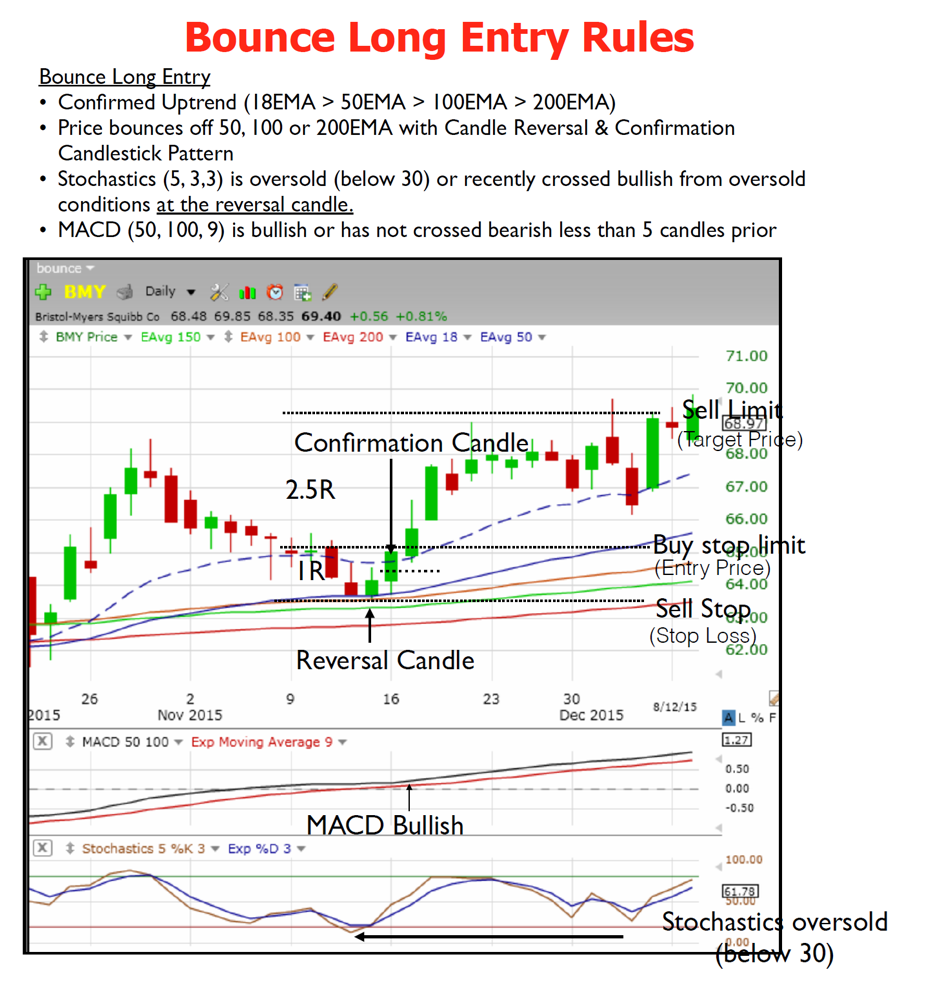# Can an Indicator be created for Bounce Long Entry?

#### mohitdas

##### New member
VIP
I was hoping to create an indicator with below condition and possibly an alert arrow at Confirmation candle for Entry and Exit along with the MACD and Stochastics to support overall conditions
• Confirmed Uptrend (18EMA > 50EMA > 100EMA > 200EMA)
• Price bounces off 50, 100 or 200EMA with Candle Reversal & Confirmation Candlestick Pattern
• Stochastics (5, 3,3) is oversold (below 30) or recently crossed bullish from oversold conditions at the reversal candle.
• MACD (50, 100, 9) is bullish or has not crossed bearish less than 5 candles priorLast edited by a moderator:

#### mohitdas

##### New member
VIP
@BenTen @horserider Do you guys mind looking at the below code when you get a chance and provide some feedback if the below code satisfies the above conditions for each MA's( 18/50/100). I was hoping to have these as Indicators, currently i use this as Scans but it very much of a hit and miss sometime i get a hit but most time hardly anything gets picked up through these scans. Please advise.

I have below code placed into three different scans which eventually saves into three different watchlist, i think it would be better if i can get this to work as an Indicator.

18 Bounce Long
MovAvgExponential("length" = 18) is greater than MovAvgExponential("length" = 50) and MovAvgExponential("length" = 50) is greater than MovAvgExponential("length" = 100) and MovAvgExponential("length" = 100) is greater than MovAvgExponential("length" = 200) and low from 1 bars ago crosses below MovAvgExponential("length" = 18) and MACDHistogramCrossover("fast length" = 18, "slow length" = 50, "crossing type" = "Negative to Positive") is true within 15 bars and MACDHistogramCrossover("fast length" = 50, "slow length" = 100, "crossing type" = "Negative to Positive") is true within 15 bars and StochasticFull("over bought" = 70, "over sold" = 30, "k period" = 5, "d period" = 3, "average type" = "EXPONENTIAL")."FullK" is less than or equal to 30 within 3 bars and open from 1 bars ago is greater than MovAvgExponential("length" = 18) and close from 1 bars ago is greater than MovAvgExponential("length" = 18) and close is greater than high from 1 bars ago and close is greater than open

50 Bounce Long
MovAvgExponential("length" = 18) is greater than MovAvgExponential("length" = 50) and MovAvgExponential("length" = 50) is greater than MovAvgExponential("length" = 100) and MovAvgExponential("length" = 100) is greater than MovAvgExponential("length" = 200) and low from 1 bars ago crosses below MovAvgExponential("length" = 50) and StochasticFull("over bought" = 70, "over sold" = 30, "k period" = 5, "d period" = 3, "average type" = "EXPONENTIAL")."FullK" is less than or equal to 30 within 3 bars and open from 1 bars ago is greater than MovAvgExponential("length" = 50) and close from 1 bars ago is greater than MovAvgExponential("length" = 50) and close is greater than high from 1 bars ago and close is greater than open

100 Bounce Long
MovAvgExponential("length" = 18) is greater than MovAvgExponential("length" = 50) and MovAvgExponential("length" = 50) is greater than MovAvgExponential("length" = 100) and MovAvgExponential("length" = 100) is greater than MovAvgExponential("length" = 200) and low from 1 bars ago crosses below MovAvgExponential("length" = 100) and StochasticFull("over bought" = 70, "over sold" = 30, "k period" = 5, "d period" = 3, "average type" = "EXPONENTIAL")."FullK" is less than or equal to 30 within 3 bars and open from 1 bars ago is greater than MovAvgExponential("length" = 100) and close from 1 bars ago is greater than MovAvgExponential("length" = 100) and close is greater than high from 1 bars ago and close is greater than open

•AriD

### Not the exact question you're looking for?

87k+ Posts
284 Online## The Market Trading Game Changer

Join 2,500+ subscribers inside the useThinkScript VIP Membership Club
• Exclusive indicators
• Proven strategies & setups
• Private Discord community
• Exclusive members-only content
• 1 full year of unlimited support

What is useThinkScript?

useThinkScript is the #1 community of stock market investors using indicators and other tools to power their trading strategies. Traders of all skill levels use our forums to learn about scripting and indicators, help each other, and discover new ways to gain an edge in the markets.

How do I get started?

We get it. Our forum can be intimidating, if not overwhelming. With thousands of topics, tens of thousands of posts, our community has created an incredibly deep knowledge base for stock traders. No one can ever exhaust every resource provided on our site.

If you are new, or just looking for guidance, here are some helpful links to get you started.

What are the benefits of VIP Membership?